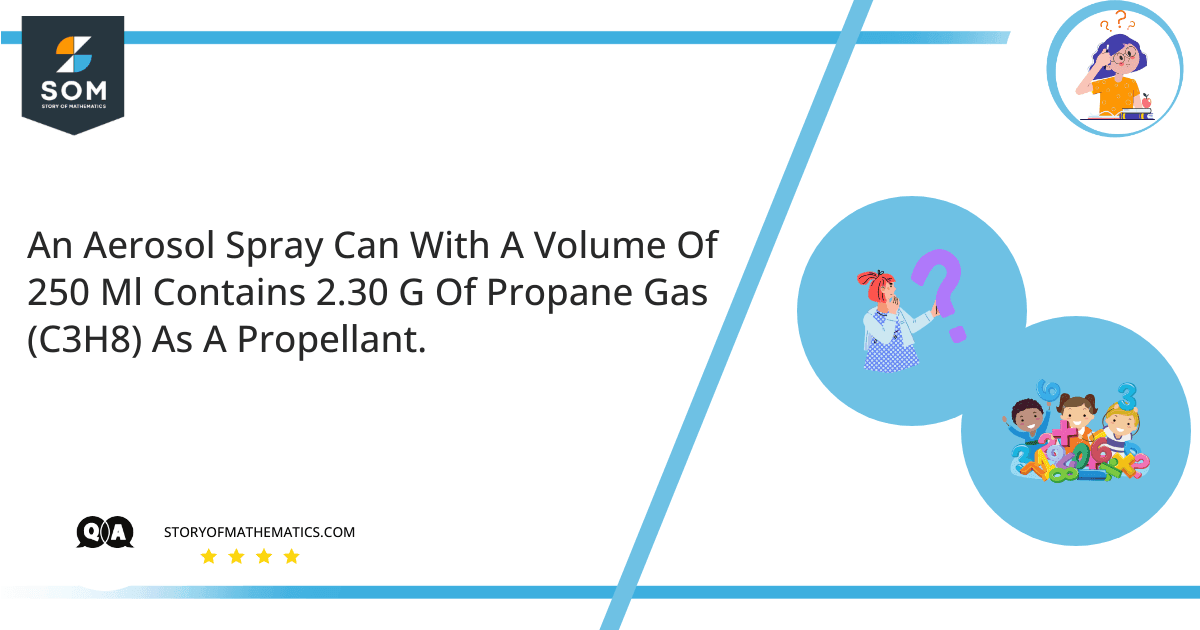# An aerosol spray can with a volume of 250 mL contains 2.30 g of propane gas (C3H8) as a propellant.-a) If the can is at $25 C$, what is the pressure in the can?
-b) What volume would the propane occupy at STP?

This question belongs to the chemistry domain and aims to explain the concepts of moles, molar mass, and Ideal gas equation. Furthermore, it explains how to calculate the volume and the pressure of the gas under any given condition.

Molar mass can be represented as “mass per mole.” It can also be explained as the sum of the mass of all the atoms in per mole of a substance.  It is expressed in units of grams per mole. Molar mass is shown for molecules or elements. In the matter of single molecules or separate atoms, the molar mass would simply be the element’s mass described in atomic mass units. Similarly, the atomic mass and the molar mass of a distinct atom are precisely equal. As molar mass and atomic mass are similar for individual atoms, molar mass can be used to estimate the particle’s uniqueness.

Mole in chemistry is a formal scientific unit for counting large portions of very small commodities such as molecules, atoms, or other defined particles.

Given Information:

Volume = $250 mL$

Mass of the propane gas = $2.30g$

The molar mass of propane gas is given as = $44.1$

Calculate the number of moles of propane gas. The formula for finding moles is given as:

$Moles \space of \space propane = \dfrac{mass}{molar \space mass}$

$\dfrac{2.30g}{44.1}$

$Moles \space of \space propane = 00522mol$

Part A

The given temperature is $25C$ which is $298K$.

The pressure can be calculated using the ideal gas equation:

$PV=nRT$

Re-arranging and making pressure $P$ the subject:

$P=\dfrac{nRT}{V}$

Inserting the values and simplifying:

$=\dfrac{(0.0522)(0.0821) (298K) }{0.250}$

$=5.11 \space atm$

Part B

The volume of propane occupied at STP can be found as follows:

$P_1 = 1 \space atm$

$T_1 = 273 \space K$

$V_1 =?$

Given Conditions:

$P_1 = 5.11 \space atm$

$T_1 = 298 \space K$

$V_1 =0.250$

$\dfrac{P_1V_1}{T_1} = \dfrac{P_2V_2}{T_2}$

Inserting the values:

$\dfrac{(1 \space atm)V_1}{273 \space K} = \dfrac{(5.11 \space atm) (0.250L)} {298 \space K}$

$\dfrac{V_1}{273 \space K} = 0.00429$

$V_1 = 0.00429 \times 273 \space K$

$V_1 = 1171 \space mL$

Part A: If the can is at $25 C$, the pressure in the can is $5.11 \space atm$.

Part B: The volume the propane occupies at STP is $1171 \space mL$.

## Example

They say that exposure to temperatures above $130 F$ may cause the can to burst. What is the pressure in the can at this temperature?

The pressure in the can at a temperature of $130 F$ is found as:

$130F$ equals to $327.4K$:

The ideal gas equation is given as:

$PV = nRT$

Re-arranging and making pressure $P$ the subject:

$P= \dfrac{nRT}{V}$

Inserting the values and simplifying:

$= \dfrac{(0.0522) (0.0821) (327.4K) }{0.250}$

$= 5.59 \space atm$